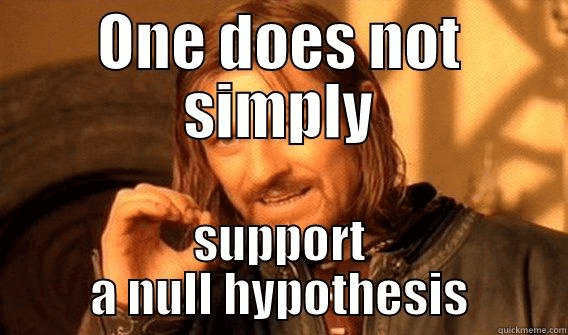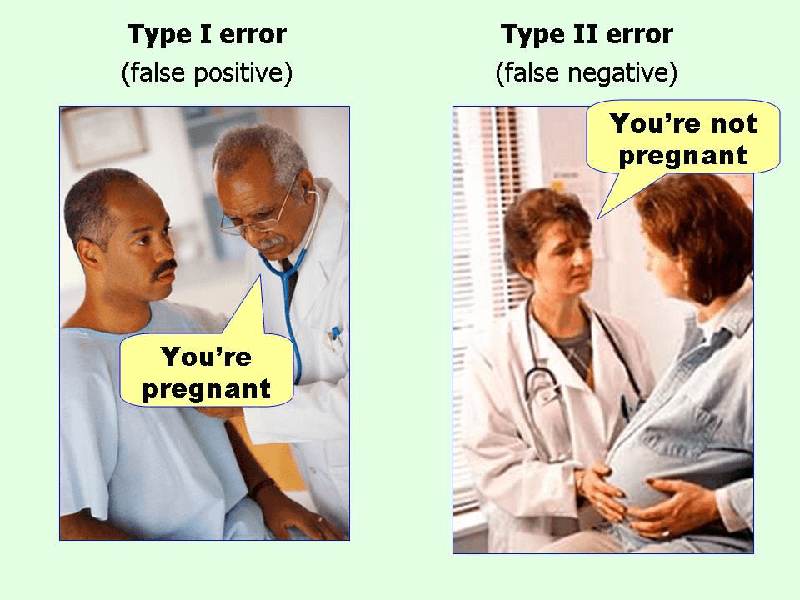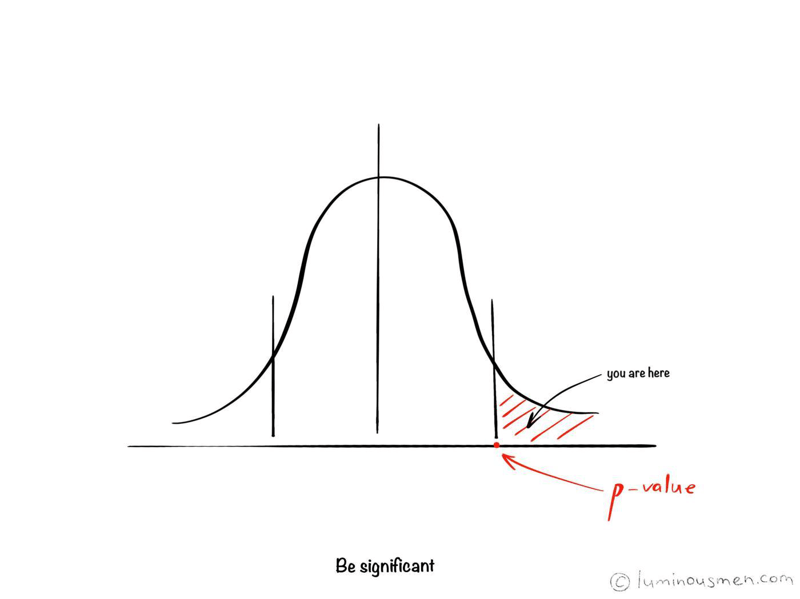There are a lot of engineers who have never been involved in statistics or data science. So, to build data science pipelines or rewrite produced by data scientists code to an adequate, easily maintained code many nuances and misunderstandings arise from the engineering side. For these Data/ML engineers and novice data scientists, I make this series of articles. I'll try to explain some basic approaches in plain English and, based on them, explain some of the Data Science model concepts.

The whole series:

## Hypothesis testing

Let us begin by getting some conceptual clarity on what a hypothesis test is. It all starts with a hypothesis.

So what is a hypothesis?

This is a question based on a specific observation that you need to prove. For a question to become a hypothesis, it must be certainly provable — this is the main condition. For example, you can prove that changing the title in an ad will increase conversion by 20%, but you won’t be able to check the question “Will changing the title help increase conversion?” In other words, the hypothesis should be specific, not vague.

Hence, the process of Hypothesis testing consists of forming questions about the data based on the gathered information and testing them using statistical methods.

Example: Testing the hypothesis that women spend more time talking on the phone than men. Suppose that 62 men and 53 women participated in the study. The average talk time was 37 minutes. per day in men and 41 minutes. per day in women. At first glance, differences are obvious, and the results confirm the hypothesis. However, such a result can be obtained by chance, even if there are no differences in the population. Therefore, the question arises — is the difference obtained in the average values sufficient to say that in general, women on average speak on the phone longer than men? What is the probability of that being true? Is this difference statistically significant?

## Null hypothesis and the alternative hypothesisThe null hypothesis is the claim about a population, which is usually formulated as the absence of differences between two populations, the absence of the influence of a factor, the absence of an effect, etc. For our example, this would be the assumption that women and men spend the same amount of time talking on the phone.

Another assumption under test (not always strictly opposite or opposite to the first) is called an alternative hypothesis. So, for the example mentioned above, it would be the following — women spend more time talking on the phone than men call.

When I started to understand the topic, I did not understand why the null hypothesis is a testable assumption, it seemed to me that the null hypothesis should be our assumption about the population (H0 changed to H1). Over time, I realized that in essence, the null hypothesis is a zero state — we assume that we do not know anything about the population. An alternative hypothesis is tested assumptions about the population, next state, next level of knowledge.

## Significance LevelsWhen testing statistical hypotheses, it is possible to make a mistake by accepting or refusing the correct hypothesis. The level of significance is the probability of making a Type I error. It's the threshold probability of an error for rejecting the null hypothesis when it is true. In other words, this is the acceptable (from the researcher point of view) probability of making a statistical error of the first type — the error that the differences are considered significant, but they are random. This probability indicated by the letter α (alpha).

I'll tell you right away about a Type II error — rejecting the null hypothesis when it is false, concluding that there is no effect, whereas it exists. The chance of a Type II error is indicated by β (beta).Usually, significance levels of 0.05, 0.01 and 0.001 are used, because the smaller the significance level, the less likely it is to make a Type I error. For example, a significance level of 0.05 means that no more than a 5% probability of error is allowed. Hence, the null hypothesis can be rejected in favor of an alternative hypothesis if, according to the results of the statistical test, the probability of error, i.e. the probability of accidental occurrence of the detected difference does not exceed 5 out of 100, i.e. there are only 5 chances out of 100 to make a mistake. If this significance level is not reached (the probability of error is above 5%), consider that the difference may be random and therefore it is impossible to reject the null hypothesis.Such a probability of error is also called a significance level and in another way a p-value. And it corresponds to the risk of a Type I error. If p <α, the null hypothesis is rejected.

Cool video for fixing who did not understand anything at all

## Choosing statistical criteria

The general idea of hypothesis testing involves:

1. State two hypotheses so that only one can be right.
2. Collecting evidence (data).
3. Formulate an analysis plan, set the criteria for a decision.
4. Physically analyze the sample data. - compute the test statistic.
5. Based on the available evidence (data) either accept or reject the null hypothesis.

Two first steps are clear — we make an assumption and gather all the needed data. The rest steps may vary based on statistical criteria.

To make decisions about which of the hypotheses (null or alternative) should be accepted, statistical criteria are used that include methods for calculating a certain indicator, based on which a decision is made to reject or accept the hypothesis, as well as the decision conditions.

For data obtained in metric scales (interval or relative) for distributions close to normal, parametric methods are used based on indicators such as mean and standard deviation. This is used to, for example, determine if samples came from the same population. In particular, the Student's t-test is used to determine the reliability of the difference in means for the two samples. Also, the F-test or analysis of variance (ANOVA) is used to understand the differences between three or more samples. Also, the ANOVA test is often used to determine the influence that independent variables have on the dependent variable in a regression study.

If the researcher is dealing with data obtained on non-metric (nominative or ordinal) scales or the samples are too small to be sure that the populations from which they are taken are based on the normal distribution, use nonparametric methods — Chi-squared test, Mann–Whitney U test, Wilcoxon signed-rank test. These methods are very simple in terms of both calculations and applying.

Choosing the statistical criteria also depend on whether the samples are independent (i.e., for example, taken from two different groups) or dependent (i.e., reflecting the results of the same group of subjects before and after exposure or after two different effects).

## Conclusion

Hypothesis testing is most often used when conducting A / B testing, as well as simply gaining new knowledge about the data.

Hypothesis testing is an essential procedure in statistics. A hypothesis test evaluates two mutually exclusive statements about a population to determine which statement is best supported by the data sample.

There are certain steps in the Hypothesis testing algorithm. But there is one that can vary - choosing statistical criteria. It's no trivial and required some knowledge of the analyzed data as well as the conditions in which criteria can be used.

Do not believe anyone but the data and test even the most obvious hypotheses. Cheers!

Previous post